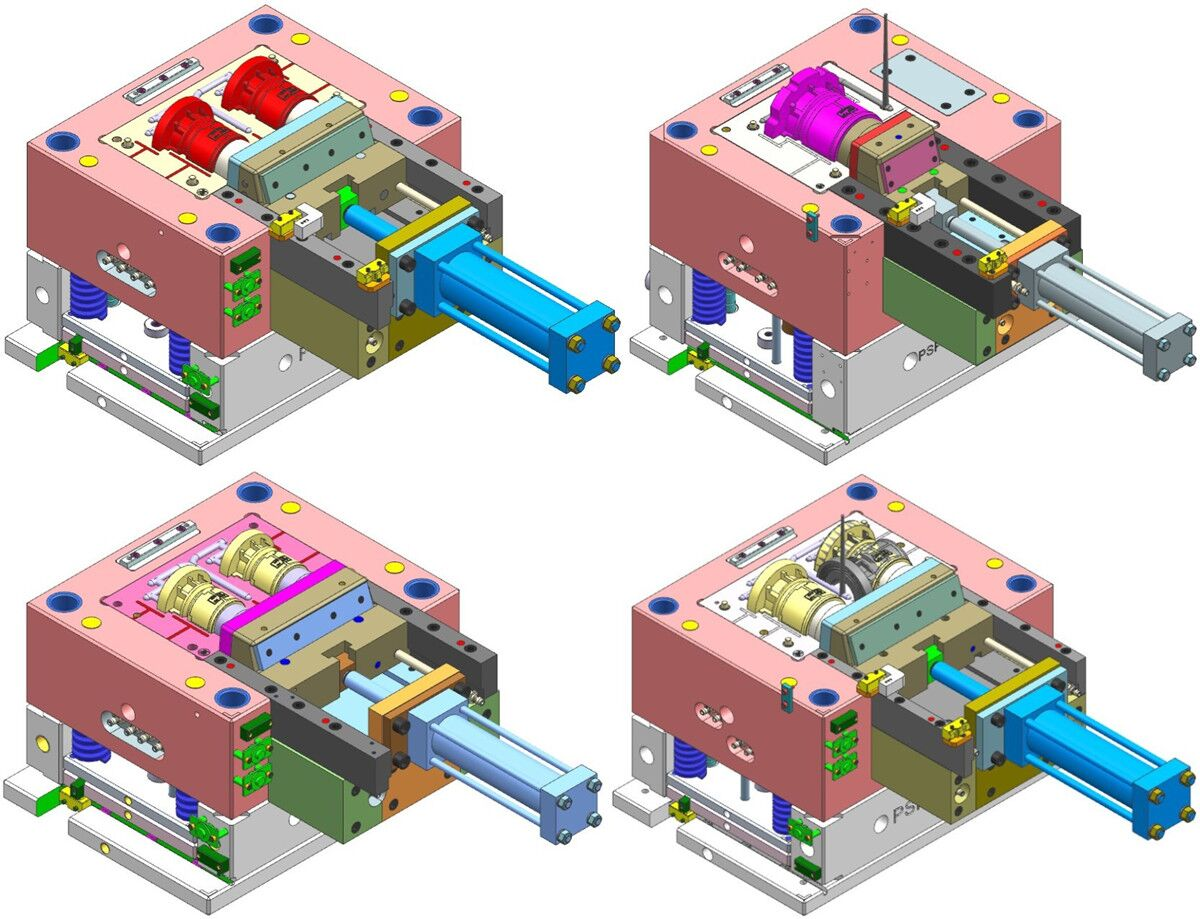# 宏程序具体计算公式及讲解，你可以看懂几个？

proe函数公式

x=50*t
y=10*sin(t*360)
z=0

r=t
theta=10+t*(20*360)
z=t*3

theta = 360 * t * 4
phi = -360 * t * 8

Rhodonea 曲线

theta=t*360*4
x=25+(10-6)*cos(theta)+10*cos((10/6-1)*theta)
y=25+(10-6)*sin(theta)-6*sin((10/6-1)*theta)
*********************************

theta=t*360
r=10+10*sin(6*theta)
z=2*sin(6*theta)

r=1
ang=360*t
s=2*pi*r*t
x0=s*cos(ang)
y0=s*sin(ang)
x=x0+s*sin(ang)
y=y0-s*cos(ang)
z=0

z=0
x = 10*t
y = log(10*t+0.0001)

rho=4
theta=t*180
phi=t*360*20

b=2.5
x=3*b*cos(t*360)+l*cos(3*t*360)
Y=3*b*sin(t*360)+l*sin(3*t*360)

a=5
x=a*(cos(t*360))^3
y=a*(sin(t*360))^3

a=10
r=a*(1+cos(theta))
theta=t*360

a=10
x=3*a*t/(1+(t^3))
y=3*a*(t^2)/(1+(t^3))

x = 4 * cos ( t *(5*360))
y = 4 * sin ( t *(5*360))
z = 10*t

x =(4 * t)
y =(3 * t) + (5 * t ^2)
z =0

r = 5
theta = t*3600
z =(sin(3.5*theta-90))+24*t

x = (a +f sin (t))cos(t)/a
y = (a -2f +f sin (t))sin(t)/b

pro/e关系式、函数的相关说明资料？

cos () 余弦
tan () 正切
sin () 正弦
sqrt () 平方根
asin () 反正弦
acos () 反余弦
atan () 反正切
sinh () 双曲线正弦
cosh () 双曲线余弦
tanh () 双曲线正切

log() 以10为底的对数
ln() 自然对数
exp() e的幂
abs() 绝对值
ceil() 不小于其值的最小整数
floor() 不超过其值的最大整数

ceil(parameter_name或number, number_of_dec_places)
floor (parameter_name 或 number, number_of_dec_places)

?可以被表示为一个数或一个使用者自定义参数。如果该参数值是一个实数，则被数控微信公号cncdar截尾成为一个整数。
?它的最大值是8。如果超过8，则不会舍入要舍入的数（第一个自变量），并使用其初值。
?如果不指定它，则功能同前期版本一样。

ceil (10.2) 值为11
floor (10.2) 值为 11

ceil (10.255, 2) 等于10.26
ceil (10.255, 0) 等于11 [ 与ceil (10.255)相同 ]
floor (10.255, 1) 等于10.2
floor (10.255, 2) 等于10.26

evalgraph(“graph_name”, x)

，其中graph_name是曲线表的名称，x是沿曲线表x-轴的值，返回y值。

trajpar_of_pnt(“trajname”, “pointname”)

?等式 – 使等式左边的一个参数等于右边的表达式。这种关系用于给尺寸和参数赋值。例如：

?比较 – 比较左边的表达式和右边的表达式。这种关系通常用于作为一个约束或用于逻辑分支的条件语句中。例如：

?特征的截面（在草绘模式中，如果最初通过选择“草绘器”>“关系”>“增加”来创建截面）。

?特征（在零件或组件模式下）。

?零件（在零件或组件模式下）。

?组件（在组件模式下）。

?组件关系 – 使用组件中的关系。如果组件包含一个或多个子组件，“组件关系”菜单出现并带有下列命令：

─当前 – 缺省时是顶层组件。
─名称 – 键入组件名。
?骨架关系 – 使用组件中骨架模型的关系（只对组件适用）。
?零件关系 – 使用零件中的关系。
?特征关系 – 使用特征特有的关系。如果特征有一个截面，那么使用者就可选择：获得对截数控微信公号cncdar面（草绘器）中截面（草绘器）中关系的访问，或者获得对作为一个整体的特征中的关系的访问。

─如果试图将截面之外的关系指派给已经由截面关系驱动的参数，则系统再生模型时给出错误信息。试图将关系指派给已经由截面之外关系驱动的参数时也同样。删除关系之一并重新生成。
─如果组件试图给已经由零件或子组件关系驱动的尺寸变量指派值时，出现两个错误信息。删除关系之一并重新生成。

─修改模型的单位元可使关系无效，因为它们没有随该模型缩放。有关修改单位的详细信息，请参阅“关于公制和非公制度量单位”帮助主题。

?尺寸符号 – 支持下列尺寸符号类型：

─d# – 零件或组件模式下的尺寸。

─d#:# – 组件模式下的尺寸。组件或组件的进程标识添加为后缀。

─rd# – 零件或顶层组件中的参考尺寸。

─rd#:# – 组件模式中的参考尺寸（组件或组件的进程标识添加为后缀）。

─rsd# – 草绘器中（截面）的参考尺寸。

─kd# – 在草绘（截面）中的已知尺寸（在父零件或组件中）。

?公差 – 这些是与公差格式相关连的参数。当尺寸由数字的转向符号的时侯出项这些符号。

─tpm# – 加减对称格式中的公差；#是尺寸数。

─tp# – 加减格式中的正公差；#是尺寸数。

─tm# – 加减格式中的负公差；#是尺寸数。

?实例数 – 这些是整数参数，是数组方向上的实例个数。

─p# – 其中#是实例的个数。

?使用者参数 – 这些可以是由增加参数或关系所定义的参数。

Volume = d0*d1*d2
Vendor = “Stockton Corp.”

─使用者参数名必须以字母开头（如果它们要用于关系的话）。
─不能使用d#、kd#、rd#、tm#、tp#、或tpm#作为使用者参数名，因为它们是由尺寸保留使用的。
─使用者参数名不能包含非字母数字字符，诸如!、@、#、\$。

x=ɑsinφ cosφ

y=ɑφsinφ

(φ2=2π+φ1)。

，即刀刃高度为零，刀刃应在x轴线上(即在通过木段回转轴线——卡轴中心线的水平面内)。也可以说，不管要求旋切单板厚度的大小如何，刀刃高度总是为零(h=0)

x=acosφ1+aφ1sinφ1

y= asinφ1-aφ1cosφ1

S=△χ[acos(2π+φ1)+a(2π+φ1)sin(2π+φ1)]-[acosφ1+acosφ1+ aφ1sinφ1]

=[acosφ1+ a(2π+φ1)sinφ1] -[acosφ1+2φ1sinφ1]

=21πasinφl

acos270°=-a=h。为了保证单板质量，在旋切加工过程中希望旋刀相对于木

h>0 此时旋切曲线近似于阿基米德螺旋线；

h=0 为阿基米德螺旋线；

0>h>-a 为伸长了的渐开线

h=-a 为渐开线；

h<-a 为缩短了的渐开线。

rho=20*t^2
theta=60*log(30)*t
phi=7200*t

“rho=200*t”
“theta=900*t”
“phi=t*90*10”

r=5+0.3*sin(t*180)+t
theta=t*360*30
z=t*5

x=50*t
y=10*sin(t*360)
z=0

r=t
theta=10+t*(20*360)
z=t*3

rho = 8 * t
theta = 360 * t * 4
phi = -360 * t * 8

Rhodonea 曲线

theta=t*360*4
x=25+(10-6)*cos(theta)+10*cos((10/6-1)*theta)
y=25+(10-6)*sin(theta)-6*sin((10/6-1)*theta)

theta=t*360
r=10+10*sin(6*theta)
z=2*sin(6*theta)

r=1
ang=360*t \90*t
s=2*pi*r*t \pi*r.t/2
x0=s*cos(ang)
y0=s*sin(ang)
x=x0+s*sin(ang)
y=y0-s*cos(ang)
z=0

z=0
x = 10*t
y = log(10*t+0.0001)

rho=4
theta=t*180
phi=t*360*20

l=2.5
b=2.5
x=3*b*cos(t*360)+l*cos(3*t*360)
Y=3*b*sin(t*360)+l*sin(3*t*360)

a=5
x=a*(cos(t*360))^3
y=a*(sin(t*360))^3

a=10
r=a*(1+cos(theta))
theta=t*360

a=10
x=3*a*t/(1+(t^3))
y=3*a*(t^2)/(1+(t^3))

x = 4 * cos ( t *(5*360))
y = 4 * sin ( t *(5*360))
z = 10*t

x =(4 * t)
y =(3 * t) + (5 * t ^2)
z =0

r = 5
theta = t*3600
z =(sin(3.5*theta-90))+24*t
３０度锥孔加工

G90G54G00X0Y0M03S2500:
G43Z50.H01M08:
Z2.
#1=0.05
WHILE[#1LE5.]DO1
#2=TAN[15.]*#1
#3=5.-#2
G01Z-#1F50
X-#3F500
G02I#3
G01X0
#1=#1+0.05
END1
G0Z50.M05
G91G28Z0Y0M09

1、变量

(1)变量的表示

(2)变量的引用

(3)变量的类型
0MC系统的变量分为公共变量和系统变量两类。
1）公共变量

2）系统变量

2、宏指令G65

m–宏程序功能，数值范围01～99；
#i–运算结果存放处的变量名；
#j–被操作的第一个变量，也可以是一个常数；
#k–被操作的第二个变量，也可以是一个常数。### 觉得文章有用就打赏一下文章作者

#### 支付宝扫一扫打赏#### 微信扫一扫打赏